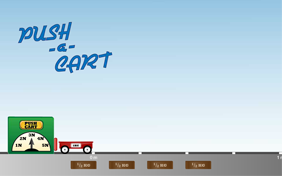# Push-A-CartGive me a push! How many times have you heard that phrase during your life? But is this saying so easy to comprehend scientifically? Moving an object sometimes requires an in-depth understanding of force, mass, acceleration, velocity, speed, distance, displacement, scalars, and vectors. Whew! That’s a lot to grasp...but that’s my push to get you started on this investigation. Start rolling!

### What is mass?

Mass is defined as the amount of matter contained in an object. The mass of an object does not change depending on its location. Mass is generally measured in units of grams or kilograms. One kilogram is equal to one thousand grams.

### What is force?

A force can simply be defined as any push or pull. However, a more in-depth definition can be applied to the phrase "net force." A net force is any push or pull that causes a change in the motion or shape of an object. If a car is standing still, a net force is needed to start the car moving. If the car's moving, a net force is required to slow it down, speed it up or to change its direction.

### What is speed?

Speed is the distance an object travels in an elapsed time. An object traveling at a higher speed will travel a greater distance in the same amount of time as an object moving at a lower speed. A common unit used to measure speed is miles per hour (mph); however, scientists usually prefer a metric measure, such as meters per second (m/s). Speed is a scalar quantity since its value can be expressed only by its magnitude or size.

### What is velocity?

Velocity is a vector relative of speed and is defined as the displacement of an object divided by the elapsed time of travel. Displacement differs from distance traveled in that displacement is how far an object is from its starting point. A race car that makes one complete lap on a one-mile long track has traveled a distance of one mile, but has a displacement of zero because the car is back to its original starting position. Since velocity is a vector, it must be defined by a size (how fast) and a direction.

### What is acceleration?

Acceleration is the rate of change of velocity over time. Simply put, it is the rate at which an object speeds up or slows down. For example, if a car is standing still and the driver speeds up to 60 miles per hour (mph), the acceleration rate is how quickly the car reached 60 mph. Acceleration also works in the reverse, which is often referred to as deceleration. If the car slows to 20 mph, the deceleration rate is how long it takes the car to slow down. Note that this is different than speed, which is how quickly an object moves over a particular distance.